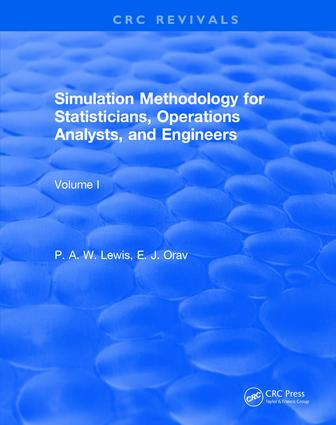# Simulation Methodology for Statisticians, Operations Analysts, and Engineers (1988)

## 1st Edition

Chapman and Hall/CRC

434 pages

##### Purchasing Options:\$ = USD
Hardback: 9781138105379
pub: 2017-10-02
SAVE ~\$54.00
\$270.00
\$216.00
x
eBook (VitalSource) : 9780203710319
pub: 2017-11-22
from \$37.48

FREE Standard Shipping!

### Description

Students of statistics, operations research, and engineering will be informed of simulation methodology for problems in both mathematical statistics and systems simulation. This discussion presents many of the necessary statistical and graphical techniques.

A discussion of statistical methods based on graphical techniques and exploratory data is among the highlights of Simulation Methodology for Statisticians, Operations Analysts, and Engineers.

For students who only have a minimal background in statistics and probability theory, the first five chapters provide an introduction to simulation.

### Table of Contents

MODELING AND CRUDE SIMULATION

Definition of Simulation

Golden Rules and Principles of Simulation

Modeling: Illustrative Examples and Problems

The Modeling Aspect of Simulation

Single-Server, Single-Input, First-In/First-Out (FIFO) Queue

Multiple-Server, Single-Input Queue

An Example from Statistics: The Trimmed t Statistic

An Example from Engineering: Reliability of Series Systems

A Military Problem: Proportional Navigation

Comments on the Examples

Crude (or Straightforward) Simulation and Monte Carlo

Introduction: Pseudo-Random Numbers

Crude Simulation

Details of Crude Simulation

A Worked Example: Passage of Ships Through a Mined Channel

Generation of Random Permutations

Uniform Pseudo-Random Variable Generation

Introduction: Properties of Pseudo-Random Variables

Historical Perspectives

Current Algorithms

Recommendations for Generators

Computational Considerations

The Testing of Pseudo-Random Number Generators

Conclusions on Generating and Testing Pseudo-Random Number Generators

SOPHISTICATED SIMULATION

Descriptions and Quantifications of Univariate Samples: Numerical Summaries

Introduction

Sample Moments

Percentiles, the Empirical Cumulative Distribution Function, and Goodness-of-Fit Tests

Quantiles

Descriptions and Quantifications of Univariate Samples: Graphical Summaries

Introduction

Numerical and Graphical Representations of the Probability Density Function

Alternative Graphical Methods for Exploring Distributions

Comparisons in Multifactor Simulations: Graphical and Formal Methods

Introduction

Graphical and Numerical Representation of Multifactor Simulation Experiments

Specific Considerations for Statistical Simulation

Summary and Computing Resources

Assessing Variability in Univariate Samples: Sectioning, Jackknifing, and Bootstrapping

Introduction

Preliminaries

Assessing Variability of Sample Means and Percentiles

Sectioning to Assess Variability: Arbitrary Estimates from Non-Normal Samples

Bias Elimination

Variance Assessment with the Complete Jackknife

Variance Assessment with the Bootstrap

Simulation Studies of Confidence Interval Estimation Schemes

Bivariate Random Variables: Definitions, Generation, and Graphical Analysis

Introduction

Specification and Properties of Bivariate Random Variables

Numerical and Graphical Analyses for Bivariate Data

The Bivariate Inverse Probability Integral Transform

Ad Hoc and Model-Based Methods for Bivariate Random Variable Generation

Variance Reduction

Introduction

Antithetic Variates: Induced Negative Correlation

Control Variables

Conditional Sampling

Importance Sampling

Stratified Sampling

### Subject Categories

##### BISAC Subject Codes/Headings:
BUS049000
BUSINESS & ECONOMICS / Operations Research
MAT000000
MATHEMATICS / General
MAT029000
MATHEMATICS / Probability & Statistics / General